# Lesson 1: Photons

1.1 Photoelectric effect – 1.2 Explanation – 1.3 Experimental verification – 1.4 Momentum – 1.5 Progress check – 1.6 Summary

The aim of the milq course is to make you familiar with the content of the Internet portal for quantum physics. You will certainly find new ideas here, but also information you recognize from your lessons.
The course starts with the photoelectric effect. If you are very familiar with it, read the Summary (Section 1.6) of the first lesson on this website just to be sure, and then proceed to “Lesson 2: Preparation”.
If you want to work through “Lesson 1: Photons” in more detail,
you can download Chapter 1 of the teaching materials as a pdf file.

### 1.1. The photoelectric effect: Light releases electrons from metal surfaces

Experiment 1.1 (Hallwachs experiment):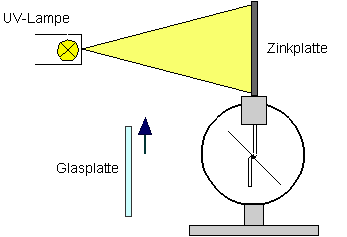Hallwachs experiment; photoelectric effect

The light-induced emission of electrons from a metal surface is called the external photoelectric effect. One example for this is the Hallwachs experiment (see diagram). A charged zinc plate is discharged by light knocking electrons out of the plate.
Attempts to explain the effect using the wave theory of light run into several difficulties. The experiment is therefore deemed to be evidence for the photon hypothesis.

### 1.2 Using photons to explain the photoelectric effect

According to the concept of the photon, light does not flow away from a light source as electromagnetic energy with a spatially continuous distribution but rather as a multitude of energy portions. In this sense we can call it a “stream of particles”.
The energy equation for the photoelectric effect is: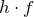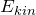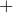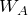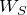Photon energy = kinetic energy of the electron + work function + energy released by collisions

The maximum energy of the electrons released is therefore: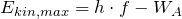(Einstein’s equation).

In the photoelectric effect, photons impinge on a metal surface and are absorbed.
The energyof a photon is transferred to an electron; the electron can leave the metal with the energy it has gained.

### 1.3 Experimental verification of the energy equation and determination of Planck’s constant

The energy equation can be checked experimentally with the retarding potential method. To carry out the experiment below with the aid of the interactive on-screen experiment (IBE), download the “IBE zum Photoeffekt-deutsch.exe“.

#### Experiment 1.3::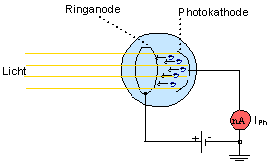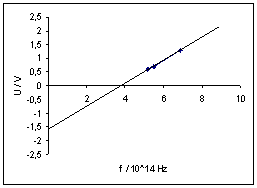Planck’s constant h can be determined from the gradient of the straight line through the measured points (precise measurements yield: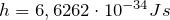).

With different materials, the straight lines obtained have the same gradient but different intercepts on the axes. This comes about because they have different, material-dependent work functions.

Determination of the work function for a metal surface

Ways of determining h experimentallyh

### 1.4 Photon momentum

The successful interpretation of the photoelectric effect suggests a particle theory for light. This is also supported by the experimental finding that photons have a momentum, which they can transfer to other particles (e. g. to an electron in the Compton effect).

A photon of frequency f has

an energy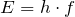and a momentum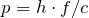.

Light exhibits particle behavior under certain circumstances (e. g. in the photoelectric effect), under other circumstances wave behavior e. g. in interference phenomena).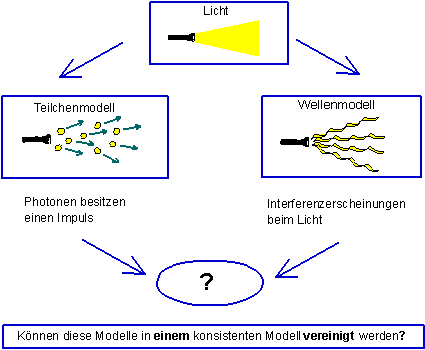Light – particle or wave?

Quantum mechanics (or quantum electrodynamics in the case of photons) naturally provides a mathematical model with which all previous experiments on quantum objects could be described correctly. A mathematical model does not facilitate a graphic understanding, however.

In the following chapters we will show that the so-called “wave-particle duality” loses much of its mysterious character with Born’s probabilistic interpretation, however.

### 1.5 Progress check

The following points were important in this chapter:

• Set up, execution, observation and interpretation of the experiments for the photoelectric effect.
• Experimental determination of Planck’s constant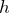.
• Photons have a momentum.

Before you move on to the next chapter, make sure you know the fundamental ideas behind these points. You can then check this with the aid of the Summary.

### 1.6 Zusammenfassung von Lektion 1: Photonen

This chapter describes the photoelectric effect with Einstein’s interpretation in more detail. Photons dislodge electrons from metal surfaces, whereby the photon transfers the energy.

The photoelectric effect can be demonstrated experimentally with a photoelectric cell or the experimental set-up for the Hallwachs effect. Planck’s constant can be determined with the aid of the retarding potential method, for example.

Photons have a momentum and can transfer it to other particles of matter (e. g. in the Compton effect). The following applies: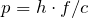.